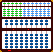THE LEE KAI-CHEN ABACUS

Little is known about Lee Kai-chen. The manuals that accompany the abacus give no information about him or how he came to invent his abacus. All remains a mystery. About the abacus he created, however, we do know a little more. During the middle part of the last century Lee produced a small number of extraordinary and unusual abaci. The Lee Kai-chen Abacus was made in Taiwan where it was manufactured for only a few years. Manuals show 1959 as the copyright date for the abacus and June and December 1958 as printing dates for the manual. Although the manuals that accompany his abaci seem to have remained largely unchanged, the abacus itself did go through a number of design modifications. Whether or not these modifications were a conscious effort to improve the abacus or whether it was a manufacturing decision is unclear. Perhaps the answer lies somewhere in between.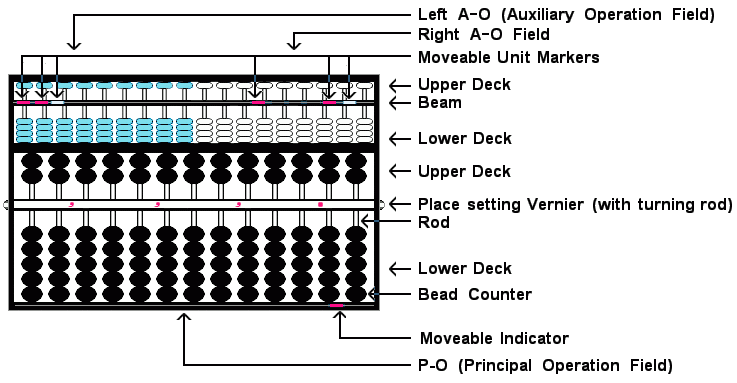A few design modifications of note.

• In the Left and Right A-O Field (Auxiliary Operations Field), early Lee's Abaci had 20 soroban rods; later this was changed to 18.

• Early Lee's Abaci had fixed unit rod markers on the beam of the soroban in the upper part of the frame. On later models, the fixed markers were replaced with six moveable unit markers.

• Early models had a wooden strut dividing left and right A-O field. Later the strut was abandoned and the two fields were divided into rods carrying different colored beads. Depending on the abacus, the 9 rods on the left-hand side had beads that were either blue or green while the nine rods on the right-hand side had beads that were either white or grey.

Three characteristics that make Lee's Abacus different from the rest:

1. In looking at Lee's abacus the first impression often is, it's made up of two abaci; a Japanese soroban above and a Chinese suan pan below. In actuality it's three abaci. Because the soroban in upper portion of the frame is divided into two sections, there are two soroban on top and one suan pan below.

2. The beam on the suan pan in the lower portion of the frame has a moveable place setting vernier. The vernier winds around two pulleys called turning rods. The vernier is marked with a dot for the decimal and with commas to signify place value (thousands, ten thousands and hundred thousands, etc.). When solving problems of division and multiplication involving decimals, Lee's techniques require the operator to make adjustments by turning the pulleys thereby adjusting the vernier to  ensure correct decimal placement. One important aspect to keep in mind when using the vernier; Lee places the decimal and commas *in between* rods. This is different from the Japanese way of doing things where unit dots on the beam of soroban are typically centered directly over the rods.

3. At very bottom of the frame there is a moveable red Indicator. This indicator is used as an aide to help locate place value in problems of multiplication and division.

## Lee Kai-chen Techniques

For both addition and subtraction, problems are solved in the principle operation field. Problems are worked out exactly as if using a normal Chinese suan pan or Japanese soroban, with one possible exception. In some cases the operator might have to make an adjustment to the vernier to ensure that the decimal point corresponds with decimal numbers in the problem. This would be the only difference. Just to recap and to become a little more familiar with the diagrams here are two simple examples, one each for addition and for subtraction.

###ADDITION

Example: 76.25 + 45.33 = 121.58

The vernier: In the P-O field, choose rod I to be the unit rod. Set the decimal on the vernier just to the right of rod I.

Step 1: Set 76.25 onto rods HIJK.  (Fig.1)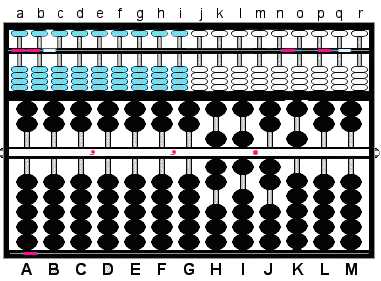Fig.1 ```Step 1 a b c d e f g h i j k l m n o p q r 0 0 0 0 0 0 0 0 0 0 0 0 0 0 0 0 0 0 ``` ```  A B C D E F G H I J K L M   , , • <==== Vernier   0 0 0 0 0 0 0 7 6.2 5 0 0 Step 1```

Step 2: Solve the addition using complementary numbers where necessary just as you would on a normal suan pan. This leaves the answer 121.58 on rods GHIJK. (Fig.2)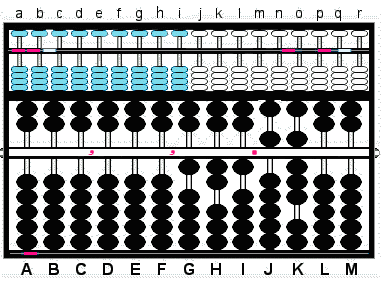Fig.2 ```Step 2 a b c d e f g h i j k l m n o p q r 0 0 0 0 0 0 0 0 0 0 0 0 0 0 0 0 0 0 ``` ```  A B C D E F G H I J K L M   , , •      0 0 0 0 0 0 0 7 6.2 5 0 0   + 4 5.3 3 Step 2   0 0 0 0 0 0 1 2 1.5 8 0 0```

###SUBTRACTION

Example: 102.43 - 67.89 = 34.54

The vernier: In the P-O field, choose rod I to be the unit rod. Set the decimal on the vernier just to the right of rod I.

Step 1: Set 102.43 onto rods GHIJK. (Fig.3)Fig.3 ```Step 1 a b c d e f g h i j k l m n o p q r 0 0 0 0 0 0 0 0 0 0 0 0 0 0 0 0 0 0 ``` ```  A B C D E F G H I J K L M   , , • <==== Vernier   0 0 0 0 0 0 1 0 2.4 3 0 0 Step 1```

Step 2: Solve the subtraction using complementary numbers where necessary just as you would on a normal suan pan. This leaves the answer 34.54 on rods HIJK. (Fig.4)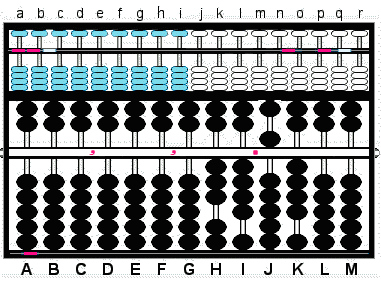Fig.4 ```Step 2 a b c d e f g h i j k l m n o p q r 0 0 0 0 0 0 0 0 0 0 0 0 0 0 0 0 0 0 ``` ```  A B C D E F G H I J K L M   , , •      0 0 0 0 0 0 1 0 2.4 3 0 0   - 6 7.8 9 Step 2 0 0 0 0 0 0 0 3 4.5 4 0 0```

###MULTIPLICATION

Japanese multiplication techniques require setting multiplier on the left and multiplicand on the right. In setting the multiplicand on the left and the multiplier on the right, Lee does the opposite. This difference aside, Lee's technique for adding products to the frame in a right-left manner is the same as one described by Takashi Kojima in his book, 'Advanced Abacus: Japanese Theory and Practice'. In the book Kojima illustrates several different methods for solving problems of multiplication. Of this method he says, "This arithmetic method is the oldest multiplication method here introduced. It was used extensively in Japan until around 1930, ..."

Basically Lee's procedure is this:

1. Set the decimal on the moveable vernier to a convenient location.
2. Set the multiplicand in the left A-O field and the multiplier in the right A-O field.
3. Add the products to rods in the P-O field.
4. The indicator at the bottom of the frame helps determine place value of the product.

**Indicator**
Where both multiplicand and  multiplier are whole numbers,
begin by placing the indicator directly under the unit rod.

Example 1:   78 x 23 = 1794

Step 1: In the left A-O field, set the multiplicand 78 on rods hi and  in the right A-O field set 23 on rods lm.

The vernier: In the P-O field, choose rod I to be the unit rod. Set the decimal on the vernier just to the right of rod I.

The indicator: Slide the indicator directly underneath unit rod I. **The indicator under rod I will assist in finding the correct number placement in the product.** (Fig.5)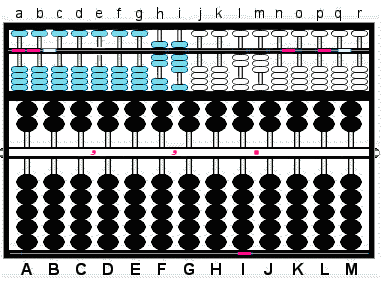Fig.5 ```Step 1 a b c d e f g h i j k l m n o p q r 0 0 0 0 0 0 0 7 8 0 0 2 3 0 0 0 0 0 Step 1 ``` ```  A B C D E F G H I J K L M   , , • <==== Vernier   0 0 0 0 0 0 0 0 0.0 0 0 0 ▬ <=== Indicator```

Step 2:
Multiply 3 on m by 8 on rod i. In the P-O field, add the product 24 to rods HI. (The '4' in 24 is added to the indicator rod.)

2a: Multiply the same 3 on m by 7 on rod h. In the P-O field, add the product 21 to rods GH. This leaves the partial product 234 on rods GHI. (Fig.6)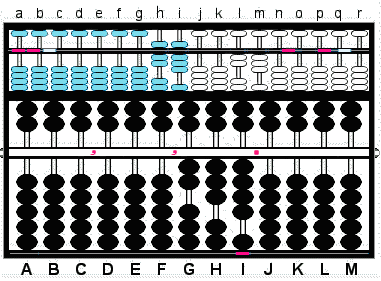Fig.6 ```Step 2 a b c d e f g h i j k l m n o p q r 0 0 0 0 0 0 0 7 8 0 0 2 3 0 0 0 0 0 ``` ```  A B C D E F G H I J K L M   , , •      0 0 0 0 0 0 0 0 0.0 0 0 0   + 2 4 Step 2 + 2 1 Step 2a 0 0 0 0 0 0 2 3 4.0 0 0 0 ▬ <=== Indicator```

The indicator:
Slide the indicator one rod to the left placing it under rod H.

Step 3: Multiply 2 on l by 8 on rod i.  In the P-O field, add the product 16 to rods GH. (This time the '6' in 16 is added to the indicator rod.)

3a & the answer: Multiply the same 2 on l by 7 on rod h. Add the product 14 to rods FG, leaving the answer 1,794 on rods FGHI. (Fig.7)Fig.7 ```Step 3 a b c d e f g h i j k l m n o p q r 0 0 0 0 0 0 0 7 8 0 0 2 3 0 0 0 0 0 ``` ```  A B C D E F G H I J K L M   , , •    0 0 0 0 0 0 2 3 4.0 0 0 0   + 1 6 Step 3 + 1 4 Step 3a 0 0 0 0 0 1,7 9 4.0 0 0 0 ▬ <=== Indicator```

### Multiplying Decimals Numbers

When multiplying pure decimal or mixed decimal numbers there are a couple of things to keep in mind:

• The placement of the indicator is governed by the decimal numbers in the multiplier.
• The placement of the vernier decimal is governed by the decimal numbers in the multiplicand.

**The Indicator**
When setting up the problem on the abacus, for every number in the decimal fraction in
the multiplier move the indicator one place to the right of the chosen unit rod

For the Multiplier:

```17.2.......Place the indicator 1 rod to the right of the chosen unit rod.
1.23.......Place the indicator 2 rods to the right of the chosen unit rod.
0.003......Place the indicator 3 rods to the right of the chosen unit rod, and so on.```

**The Vernier Decimal **
After completing the multiplication, for every decimal number in the
multiplicand move the vernier decimal  one place to the left of the chosen unit rod.

For the Multiplicand:

```35.4.......Move the decimal vernier 1 rod to the left of the chosen unit rod.
5.78.......Move the decimal vernier 2 rods to the left of the chosen unit rod.
0.005......Move the decimal vernier 3 rods to the left of the chosen unit rod, and so on.
```

Example 1: 4.5 x 7.003 = 31.5135

Step 1: In the left A-O field, set the multiplicand 4.5 on rods hi. In the right A-O field, set 7.003 on rods lmno.

The moveable unit markers: In both the left and right A-O fields slide the unit markers to separate the whole and decimal numbers.

The vernier: In the P-O field, choose rod G to be the unit rod. Set the decimal on the vernier just to the right of rod G.

The indicator: Because there are three decimal numbers in the multiplier, slide the indicator three rods to the right of unit rod G. Place it under rod J. **Once again, the indicator under rod J will assist in finding the correct number placement in the product.**  (Fig.8)Fig.8 ```Step 1 a b c d e f g h.i j k l.m n o p q r 0 0 0 0 0 0 0 4.5 0 0 7.0 0 3 0 0 0 Step 1 ``` ```  A B C D E F G H I J K L M   , , • <===== Vernier   0 0 0 0 0 0 0.0 0 0 0 0 0 ▬ <=== Indicator```

Step 2:
Multiply 3 on rod o by 5 on rod i. In the P-O field, add the product 15 to rods IJ. (The '5' in 15 is added to the indicator rod.)

2a: Multiply the same 3 on rod o by 4 on rod h. In the P-O field, add the product 12 to rods HI. This leaved the partial product 135 on rods HIJ. (Fig.9)Fig.9 ```Step 2 a b c d e f g h.i j k l.m n o p q r 0 0 0 0 0 0 0 4.5 0 0 7.0 0 3 0 0 0 ``` ```  A B C D E F G H I J K L M   , , •   0 0 0 0 0 0 0.0 0 0 0 0 0 + 1 5 Step 2 + 1 2 Step 2a   0 0 0 0 0 0 0.1 3 5 0 0 0 ▬ <=== Indicator```

The indicator:
The next number to be multiplied in the right A-O field is the multiplier 7 on rod l. Because it's three places to the left, slide the indicator three rods to the left placing it under rod G.

Step 3:  Multiply 7 on l by 5 on rod i. In the P-O field, add the product 35 to rods FG. (The '5' in 35 is added to the indicator rod.)

3a: Multiply the same 7 on rod l by 4 on h. In the P-O field, add the product 28 to rods EF.

The vernier & the answer: Because there's one decimal number in the multiplicand, adjust the vernier decimal by moving it one place to the left. This changes the unit rod from rod G to rod F and leaves the correct answer 31.5135 on rods EFGHIJ. (Fig.10)Fig.10 ```Step 3 a b c d e f g h.i j k l.m n o p q r 0 0 0 0 0 0 0 4.5 0 0 7.0 0 3 0 0 0 ``` ```  A B C D E F G H I J K L M   , , • <==== Vernier   0 0 0 0 0 0.0 1 3 5 0 0 0 + 3 5 Step 3 + 2 8 Step 3a   0 0 0 0 3 1.5 1 3 5 0 0 0 ▬ <=== Indicator```

###DIVISION

Similar to the technique for solving problems of multiplication, Lee works the numbers in the P-O field from right to left. In this case he subtracts products from the frame in a right to left manner and uses the indicator at the bottom of the frame as a guide.

Basically the procedure is this:

1. Set the decimal on the moveable vernier to a convenient location.
2. Set the divisor on the left A-O field.
3. Set the dividend in the P-O field.
4. The quotient is then formed in the right A-O field.
5. The indicator at the bottom of the frame helps determine place value of the quotient.

**Indicator**
Where dividend and  divisor are both whole numbers,
Begin by placing the indicator immediately under the rod representing
the highest order of the quotient. (Probably best explained by example.)

Example 1: 1222 ÷ 26 = 47

Step 1: In the left A-O field, set divisor 26 on rods hi.

The moveable unit markers: In the right A-O fields, choose rod m to carry the unit number in the quotient. Slide a unit marker between rods mn .

The vernier: In the P-O field, choose rod I to be the unit rod. Set the decimal on the vernier just to the right of rod I.

1b: Set the dividend 1222 on rods FGHI.

The indicator: A quick look at the problem tells us that the first step will be dividing 26 into the 122 on rods FGH. To reflect this and to help us keep track of the quotient, slide the indicator underneath rod H. (Fig.11)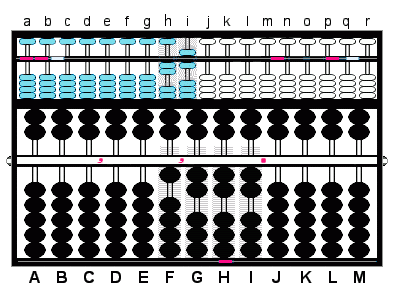Fig.11 ```Step 1 a b c d e f g h i j k l m.n o p q r 0 0 0 0 0 0 0 2 6 0 0 0 0.0 0 0 0 0 Step 1``` ```  A B C D E F G H I J K L M   , , • <==== Vernier   0 0 0 0 0 1 2 2 2.0 0 0 0 Step 1b ▬ <=== Indicator```

Step 2: Divide 26 on rods hi into 122 on rods FGH. **This is where the indicator assists in finding the correct placement for numbers in the quotient.**  The indicator is under rod H. Rod H is one rod to the left of unit rod I. Therefore, in the right A-O field, set the quotient one rod to the left of unit rod m. Set quotient 4 on rod l.

2a: Multiply quotient 4 on l by 6 on rod i and subtract the product 24 from rods GH in the P-O field.

2a: Multiply the same quotient 4 by 2 on h and subtract the product 08 from rods FG in the P-O field. This leaves the partial quotient 4 on rod l and the remainder 182 on rods GHI. (Fig.12)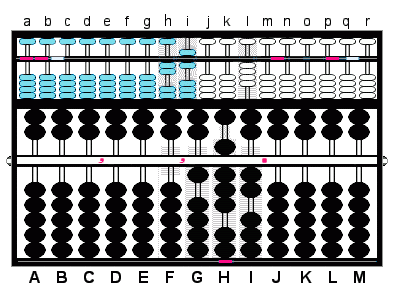Fig.12 ```Step 2 a b c d e f g h i j k l m.n o p q r 0 0 0 0 0 0 0 2 6 0 0 4 0.0 0 0 0 0 Step 2``` ```  A B C D E F G H I J K L M   , , •   0 0 0 0 0 1 2 2 2.0 0 0 0 - 2 4 Step 2a   0 0 0 0 0 0 9 8 2.0 0 0 0 - 0 8 Step 2b   0 0 0 0 0 0 1 8 2.0 0 0 0 ▬ <=== Indicator```

The indicator: Slide the indicator one rod to the right placing it under rod I.

Step 3: Divide 26 on rods hi into 182 on rods FGH. Set the quotient 7 on rod m in the right A-O field.

3a: Multiply quotient 7 by 6 on rod i and subtract the product 42 from rods HI in the P-O field.

3b & the answer: Multiply the same quotient 7 by 2 on h and subtract the product 14 from rods GH in the P-O field. This finishes the problem and leaves quotient 47 on rods lm in the right A-O field.Fig.13 ```Step 3 a b c d e f g h i j k l m.n o p q r 0 0 0 0 0 0 0 2 6 0 0 4 7.0 0 0 0 0 Step 3``` ```  A B C D E F G H I J K L M   , , •   0 0 0 0 0 0 1 8 2.0 0 0 0 - 4 2 Step 3a   0 0 0 0 0 0 1 4 0.0 0 0 0 - 1 4 Step 3b   0 0 0 0 0 0 0 0 0.0 0 0 0 ▬ <=== Indicator```

### Dividing Decimals Numbers

Lee's Abacus makes dividing decimals very easy. The only possible adjustment one might have to make is to the moveable vernier decimal. This only occurs when there are decimal numbers in the divisor.

**Decimals in the Divisor**
When setting up problems that have decimal numbers in the divisor:
for every decimal in the divisor move the vernier decimal one place to the right of  unit rod.

In this first example we'll divide a decimal number by a whole number. Because the divisor is a whole number, no adjustment to the vernier will be necessary.

Example 1: 0.0016 ÷ 8 = 0.0002

The moveable unit markers: In the right A-O field, choose rod k to be the unit rod for quotient. Slide a unit marker between rods kl.

Step 1: In the left A-O field, set divisor 8 on rod i.

The vernier: In the P-O field, choose rod G to be the unit rod. Set the decimal on the vernier just to the right of rod G.

1b: Set the dividend 0.0016 on rods GHIJK.

The indicator: Simplify the problem and think of it as dividing 8 into the 16. Slide the indicator underneath rod K.  (Fig.14)Fig.14 ```Step 1 a b c d e f g h i j k.l m n o p q r 0 0 0 0 0 0 0 0 8 0 0.0 0 0 0 0 0 0 Step 1``` ```  A B C D E F G H I J K L M   , , • <==== Vernier   0 0 0 0 0 0 0.0 0 1 6 0 0 Step 1b ▬ <=== Indicator```

Step 2: Divide 8 on rod i into 16 on rods JK. **This is where the indicator assists in finding the correct decimal place in the quotient.** The indicator is under rod K. Rod K is four rods to the right of unit rod G. Therefore, in the right A-O field, we must follow suit and set the quotient number four rods to the right of unit rod k. Set quotient 2 on rod o.

2a & the answer: Multiply quotient 2 on o by 8 on rod i and subtract the product 16 from rods JK in the P-O field. This leaves the answer 0.0002 on rods klmno in the right A-O field .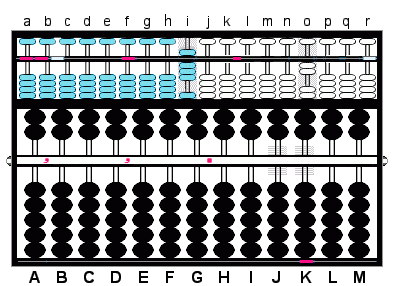Fig.15 ```Step 2 a b c d e f g h i j k.l m n o p q r 0 0 0 0 0 0 0 0 8 0 0.0 0 0 2 0 0 0 Step 2``` ```  A B C D E F G H I J K L M   , , •   0 0 0 0 0 0 0.0 0 1 6 0 0 - 1 6 Step 2a   0 0 0 0 0 0 0.0 0 0 0 0 0 ▬ <=== Indicator```

Example 2: 0.0000384 ÷ 0.0032 = 0.012

The moveable unit markers: In the left A-O field, choose rod e to be the unit rod for the divisor Slide a marker between rods ef. In the right A-O field choose rod K to be the unit rod for the quotient. Slide a marker between rods kl.

Step 1: In the left A-O field, set divisor 0.0032 on rods efghi.

The vernier: In the P-O field, choose rod D to be the unit rod. Set the decimal on the vernier just to the right of rod D.

1a: Set the dividend 0.0000384 on rods DEFGHIJK. (Fig.16)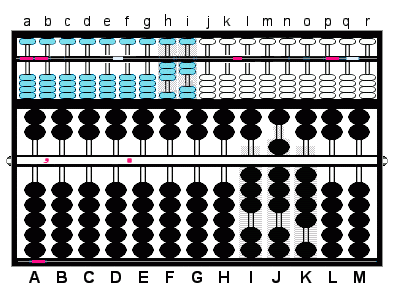Fig.16 ```Step 1 a b c d e.f g h i j k.l m n o p q r 0 0 0 0 0.0 0 3 2 0 0.0 0 0 0 0 0 0 Step 1``` ```  A B C D E F G H I J K L M   , • <==== Vernier   0 0 0 0.0 0 0 0 3 8 4 0 0 Step 1a ▬ <=== Indicator```

Step 2:

The vernier: Because the divisor has four decimal numbers, move the decimal on the vernier four rods to the right. This places it just to the right of rod H.  Rod H has become the unit rod.

The indicator: Simplify this first part of the problem and think of it as dividing 32 on rods hi into 38 on rods IJ. Slide the indicator underneath rod J. (Fig.17)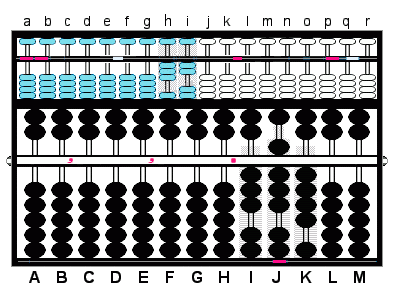Fig.17 ```Step 2 a b c d e.f g h i j k.l m n o p q r 0 0 0 0 0.0 0 3 2 0 0.0 0 0 0 0 0 0``` ```  A B C D E F G H I J K L M   , , • <==== Vernier   0 0 0 0.0 0 0 0 3 8 4 0 0 ▬ <=== Indicator```

Step 3: Divide 32 on rods hi into 38 on rods IJ. **The indicator under rod J will assist in finding the correct decimal place in the quotient.** The indicator is under rod J. Rod J is two rods to the right on unit rod H. Therefore, in the right A-O field, set the quotient two rods to the right of unit rod K.  Set quotient 1 on rod m.

3a: Multiply the quotient 1 on rod m by 2 on rod i and subtract the product 02 from rods IJ in the P-O field.

3b Multiply the same quotient 1 on rod m by 3 on rod h and subtract the product 03 from rods HI in the P-O field. This leaves the partial quotient 1 on rod m and the remainder 64 on rods JK. (Fig.18)Fig.18 ```Step 3 a b c d e.f g h i j k.l m n o p q r 0 0 0 0 0.0 0 3 2 0 0.0 1 0 0 0 0 0 Step 3``` ```  A B C D E F G H I J K L M   , , •   0 0 0 0 0 0 0 0.3 8 4 0 0 - 0 2 Step 3a - 0 3 Step 3b 0 0 0 0 0 0 0 0.0 6 4 0 0 ▬ <=== Indicator```

The indicator: Slide the indicator one rod to the right placing it under rod K.

Step 4: Divide 32 on rods hi into 64 on rods JK. Set quotient 2 on rod n.

4a: Multiply the quotient 2 on rod n by 2 on i and subtract the product 04 from rods JK.

4b & the answer: Multiply the same quotient 2 on rod n by 3 on h and subtract the product 06 from rods IJ. This finishes the problem and leaves the quotient 0.012 on rods klmn.Fig.19 ```Step 4 a b c d e.f g h i j k.l m n o p q r 0 0 0 0 0.0 0 3 2 0 0.0 1 2 0 0 0 0 Step 4``` ```  A B C D E F G H I J K L M   , , •   0 0 0 0 0 0 0 0.0 6 4 0 0 - 0 4 Step 4a - 0 6 Step 4b 0 0 0 0 0 0 0 0.0 0 0 0 0 ▬ <=== Indicator```

Lee's abacus is a lot of fun to operate. Unlike it's more basic soroban/suan pan cousins, it has lots of little moving parts which make things very interesting. The techniques are easy to follow and fun to learn. An abacus of this type might be a good instrument for school children. For teaching place value it has clear advantages. Because Lee's abacus consists of 3 separate frames this would be a very handy tool for doing more advanced calculations or for storing numbers interim. Having said this, when it comes solving basic arithmetical problems, in the hands of a good operator a simple soroban or suan pan will always be much faster and much more efficient.  - T.P.H March, 2005

Readers often email me asking questions about buying a Lee Kai-chen abacus. Since they are no longer manufactured those looking to find one are faced with a difficult task. Even though they rarely come up for auction doing an Ebay search is probably the best way to locate one. Many of Lee's abaci are in the hands of collectors.

 REFERENCES: Lee Kai-chen A Revolution of Chinese Calculators, How to learn Lee's Abacus Copyright, May, 1959 by Lee's Abacus Correspondence School Taipei, Taiwan, China First Printing, June, 1958 : Second Printing, December, 1958 Kojima, Takashi. Advanced Abacus: Japanese Theory and Practice Tokyo: Charles E. Tuttle, 1963. pgs. 36, 37, 38 Heffelfinger, Totton & Flom, Gary. Abacus: Mystery of the bead http://totton.idirect.com/abacus/ GRAPHIC IMAGES: Morris, Elizabeth Toronto, Ontario, Canada http://llizard.etherwork.net/gifs/abacus.html & Heffelfinger, Totton Toronto, Ontario, Canada PHOTOGRAPHS Heffelfinger, Totton: Toronto, Ontario, Canada ▪ Print Lee's Abacus (1.98 mb pdf)

#####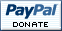Please remember these pages in your prayers :)